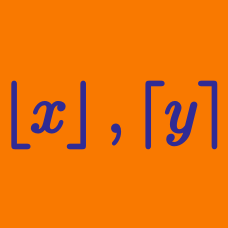Algebra

# Floor and Ceiling Functions: Level 2 Challenges

$\lfloor1\rfloor\times\lfloor2\rfloor\times\lfloor3\rfloor\times\lfloor4\rfloor\times\cdots \times\lfloor100\rfloor$

Find the trailing number of zeros of the product above.



Notation: $\lfloor \cdot \rfloor$ denotes the floor function.

Find the number of trailing zeros in the following expression.

$1!^2 \times 2!^2 \times \cdots \times 30!^2.$

If $x$ is an irrational number, what is the value of $\left \lceil \left \{ x \right \} \right \rceil$?

Notations:

True or false:

$\quad$ For any real number $x$, the value of $\{x\}$ can never be 1.

 Notation: $\{ \cdot \}$ denotes the fractional part function.

Let $3^a$ be the highest power of 3 that divides $1000!$. What is $a$?

Note: $1000! = 1 \times 2 \times 3 \ldots \times 999 \times 1000$.

×